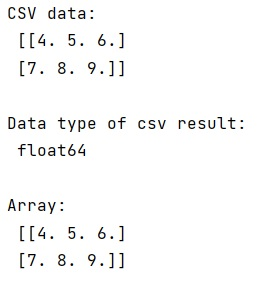# Load CSV into 2D matrix with NumPy for plotting

Learn, how to load CSV into 2D matrix with NumPy for plotting in Python?
Submitted by Pranit Sharma, on January 14, 2023

NumPy is an abbreviated form of Numerical Python. It is used for different types of scientific operations in python. Numpy is a vast library in python which is used for almost every kind of scientific or mathematical operation. It is itself an array which is a collection of various methods and functions for processing the arrays.

CSV files or Comma Separated Values files are plain text files but the format of CSV files is tabular. As the name suggests, in a CSV file, each specific value inside the CSV file is generally separated by a comma. The first line identifies the name of a data column. The further subsequent lines identify the values in rows.

Col_1_value, col_2_value , col_3_value
Row1_value1 , row_1_value2 , row_1_value3
Row1_value1 , row_1_value2 , row_1_value3

Here, the separator character (,) is called a delimiter. There are some more popular delimiters. E.g.: tab(\t), colon (:), semi-colon (;) etc.

To load a CSV file into a 2-dimensional NumPy array, we will use the numpy.loadtxt() method where we will open the CSV file and finally we will convert this data into a numpy array using the numpy array object.

Let us understand with the help of an example,

## Python code to load CSV into 2D matrix with NumPy for plotting

```# Import numpy
import numpy as np

data = np.loadtxt(open("arr.csv", "rb"), delimiter=",", skiprows=1)

# Display data
print("CSV data:\n",data,"\n")

# Display data type of csv result
print("Data type of csv result:\n",data.dtype,"\n")

# Converting data into numpy array
arr = np.array(data)

# Print array
print("Array:\n",arr,"\n")
```

Output:What's New (MCQs)

Top Interview Coding Problems/Challenges!

IncludeHelp's Blogs

Languages: » C » C++ » C++ STL » Java » Data Structure » C#.Net » Android » Kotlin » SQL
Web Technologies: » PHP » Python » JavaScript » CSS » Ajax » Node.js » Web programming/HTML
Solved programs: » C » C++ » DS » Java » C#
Aptitude que. & ans.: » C » C++ » Java » DBMS
Interview que. & ans.: » C » Embedded C » Java » SEO » HR
CS Subjects: » CS Basics » O.S. » Networks » DBMS » Embedded Systems » Cloud Computing
» Machine learning » CS Organizations » Linux » DOS
More: » Articles » Puzzles » News/Updates